# matplotlib绘图优化-绘制华夫饼图 (PyWaffle)2021年1月21日07:06:42

4923字阅读16分24秒

## 简介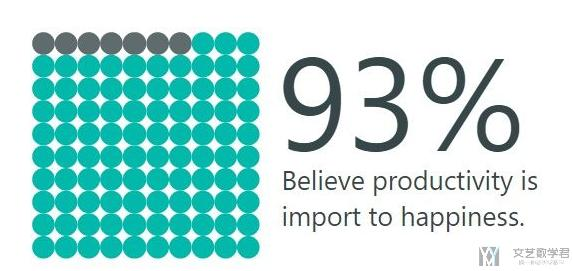## 绘制华夫饼图介绍

1. import matplotlib.pyplot as plt
2. from pywaffle import Waffle

### 绘制基础的华夫饼图

1. fig = plt.figure(
2.     FigureClass=Waffle,
3.     rows=5,
4.     columns=10,  # Either rows or columns could be omitted
5.     values=[30, 60, 10]
6. )
7. fig.show()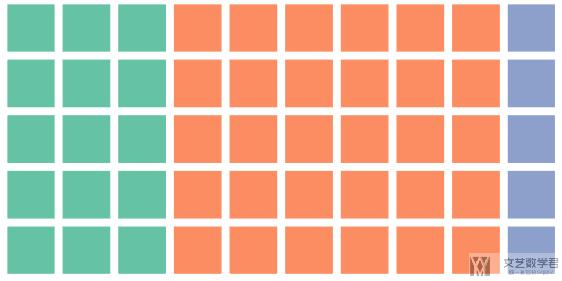### 绘制华夫饼中数值缩放

1. fig = plt.figure(
2.     FigureClass=Waffle,
3.     rows=10,
4.     columns=10,
5.     values=[60, 120, 20]
6. )
7. fig.show()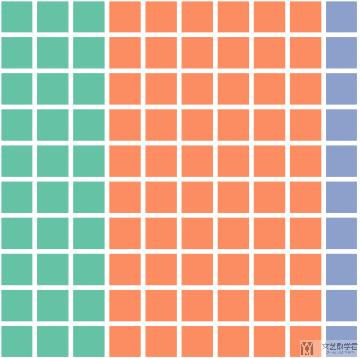### 设置华夫饼的标题和图例

1. data = {'男生':120, '女生':80}
2. fig = plt.figure(
3.     FigureClass=Waffle,
4.     rows=10,
5.     columns=10,
6.     values=data,
7.     title={
8.         'label': '男生女生的人数比例',
9.         'loc': 'center',
10.         'fontdict': {
11.             'fontsize': 20
12.         }
13.     },
14.     legend={
15.         'labels': [f"{k} ({100*v/sum(list(data.values()))}%)" for k, v in data.items()],
16.         'loc': 'lower left',
17.         'bbox_to_anchor': (0, -0.2),
18.         'ncol': 2,
19.         'framealpha': 0,
20.         'fontsize': 12
21.     }
22. )
23. fig.show()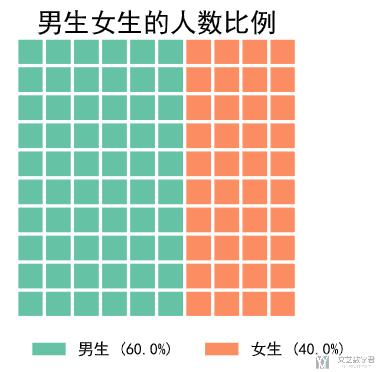### 修改 Block 的颜色

1. fig = plt.figure(
2.     FigureClass=Waffle,
3.     rows=5,
4.     columns=10,
5.     values=[30, 16, 4],
6.     colors=["#232066", "#983D3D", "#DCB732"]
7. )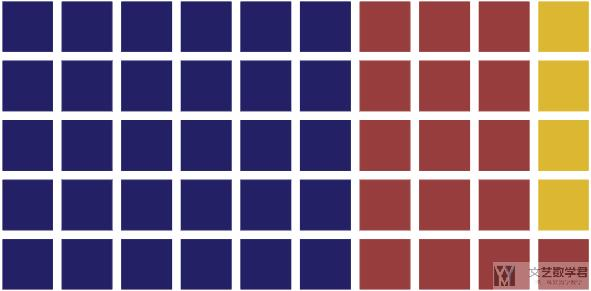1. fig = plt.figure(
2.     FigureClass=Waffle,
3.     rows=5,
4.     columns=10,
5.     values=[30, 16, 4],
6.     cmap_name="tab10"
7. )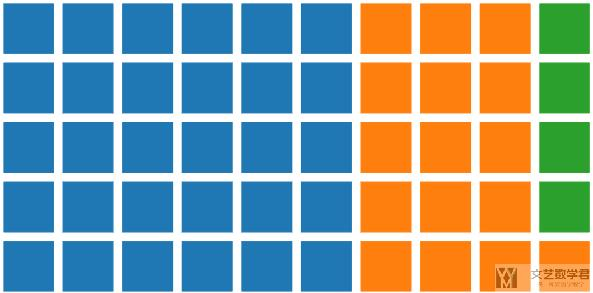### Block 的一些属性

#### 自定义 Block 的形状

1. fig = plt.figure(
2.     FigureClass=Waffle,
3.     columns=10,
4.     values=[30, 16, 4],
5.     icons='star'
6. )
7. fig.show()#### 修改 Block 之间的间距

1. fig = plt.figure(
2.     FigureClass=Waffle,
3.     rows=5,
4.     values=[30, 16, 4],
5.     interval_ratio_x=1,
6.     interval_ratio_y=0.1
7. )
8. fig.show()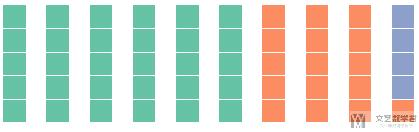#### 修改绘制方向和开始位置

1. fig = plt.figure(
2.     FigureClass=Waffle,
3.     rows=5,
4.     values=[30, 16, 4],
5.     vertical=True,
6.     starting_location='SE'
7. )
8. fig.show()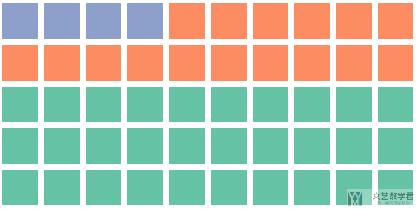#### 每一个列别都有一个新的line

1. fig = plt.figure(
2.     FigureClass=Waffle,
3.     columns=10,
4.     values=[30, 16, 4],
5.     vertical=True,
6.     block_arranging_style='new-line'
7. )
8. fig.show()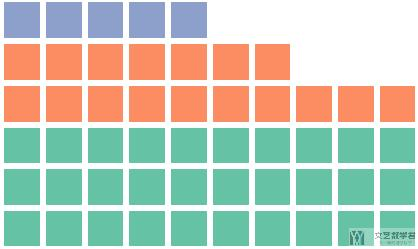### 华夫饼子图绘制

1. import pandas as pd
2. data = pd.DataFrame(
3.     {
4.         'labels': ['Hillary Clinton', 'Donald Trump', 'Others'],
5.         'Virginia': [1981473, 1769443, 233715],
6.         'Maryland': [1677928, 943169, 160349],
7.         'West Virginia': [188794, 489371, 36258],
8.     },
9. ).set_index('labels')

1. fig = plt.figure(
2.     FigureClass=Waffle,
3.     plots={
4.         '311': {
5.             'values': data['Virginia'] / 30000,
6.             'labels': [f"{k} ({v})" for k, v in data['Virginia'].items()],
7.             'legend': {
8.                 'loc': 'upper left',
9.                 'bbox_to_anchor': (1.05, 1),
10.                 'fontsize': 8
11.             },
12.             'title': {
13.                 'label': '2016 Virginia Presidential Election Results',
14.                 'loc': 'left'
15.             }
16.         },
17.         '312': {
18.             'values': data['Maryland'] / 30000,
19.             'labels': [f"{k} ({v})" for k, v in data['Maryland'].items()],
20.             'legend': {
21.                 'loc': 'upper left',
22.                 'bbox_to_anchor': (1.2, 1),
23.                 'fontsize': 8
24.             },
25.             'title': {
26.                 'label': '2016 Maryland Presidential Election Results',
27.                 'loc': 'left'
28.             }
29.         },
30.         '313': {
31.             'values': data['West Virginia'] / 30000,
32.             'labels': [f"{k} ({v})" for k, v in data['West Virginia'].items()],
33.             'legend': {
34.                 'loc': 'upper left',
35.                 'bbox_to_anchor': (1.3, 1),
36.                 'fontsize': 8
37.             },
38.             'title': {
39.                 'label': '2016 West Virginia Presidential Election Results',
40.                 'loc': 'left'
41.             }
42.         },
43.     },
44.     rows=5,
45.     colors=("#2196f3", "#ff5252", "#999999"),  # shared parameter among subplots
46.     figsize=(9, 5)  # figsize is a parameter of plt.figure
47. )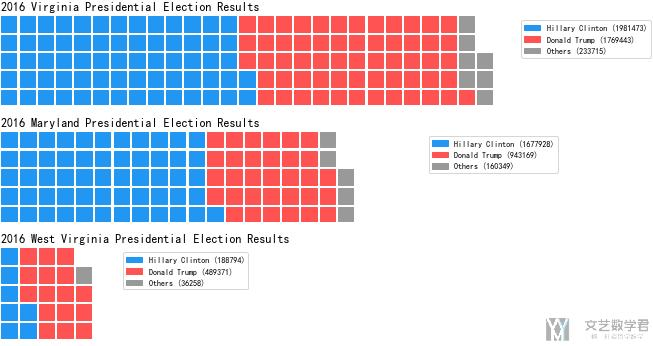## 绘制华夫饼的一些问题

### 中文的正常显示

1. from pylab import * # 设置显示中文
2. mpl.rcParams['font.sans-serif'] = ['SimHei']
3. plt.rcParams['axes.unicode_minus']=False #用来正常显示负号

• 微信公众号
• 关注微信公众号
•• QQ群
• 我们的QQ群号
•• 本文由 发表于 2021年1月21日07:06:42
• 转载请务必保留本文链接：https://mathpretty.com/13087.html